# Boost 3.3V to 5V with tiny audio amplifier

## Texas Instruments LM4871 LP3961

Wayne Rewinkel

EDN

This charge-pump circuit quietly converts a 3.3 V source to 5 V at 500 mA (Figure 1 and Figure 2). LM4871LD power amplifier makes this design idea both possible and practical, thanks to its low output resistance, low cost, compact size, and high dissipation capability. Its output resistance has an average value of 0.6 Ω: 0.5 Ω to ground and 0.7 Ω to VIN. Because it is a CMOS IC, each output can swing to its rail, limited only by the resistance of the output transistor. The leadless lead-frame package has a footprint smaller than an SO-8 but provides a θJA of 56 °C/W when soldered to a board with 1 sq in. of 1-oz copper exposed. This high thermal conductivity couples with low output resistance to allow the LM4871 to continuously deliver nearly 1 A from each of its two outputs while operating at its full rated ambient temperature of 85 °C. Internal thermal shutdown protects the device from overloads, and a shutdown pin allows you to power down the device to less than 1 µA.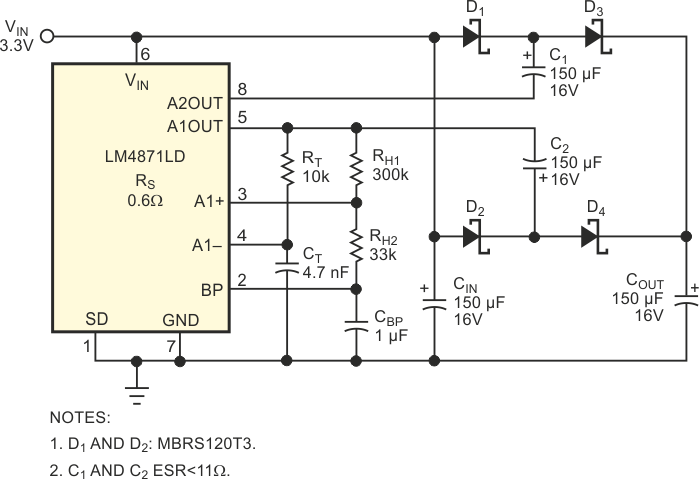Figure 1. You can use a tiny audio amplifier to boost 3.3 V to 5 V with respectable current capability.

Figure 2 shows the full circuit schematic, including the equivalent internal components. Amplifier IC1 is configured as an RC oscillator similar to a 555 timer. RT charges CT to the voltage set by the resistor divider RH1 and RH2, causing the amplifier to switch states, aided by the positive feedback from the RH resistors. The remaining internal feedback and biasing resistors connected to IC2 configure it as a simple inverter with bias at mid-supply. The amplifier outputs switch rail-to-rail out of phase with a 50% duty cycle at a frequency approximated by the following equation: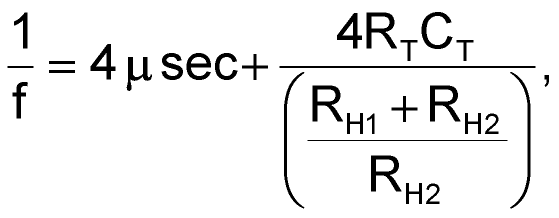f = 44 to 53 kHz.

You can calculate the output voltage across COUT from the following equation: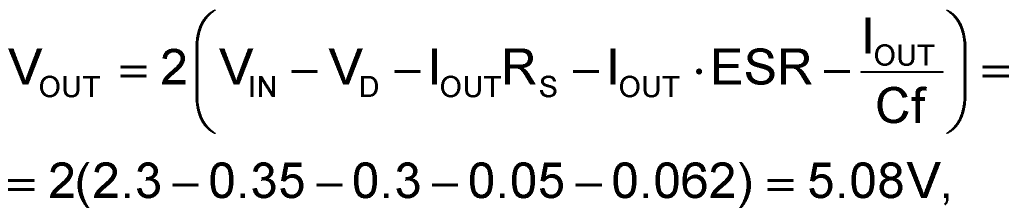where

IOUT is the average output current,
VD is the diode voltage drop at IOUT,
RS is the source resistance of IC1 and IC2,
ESR is that of C1 and C2,
C is the value of C1=C2.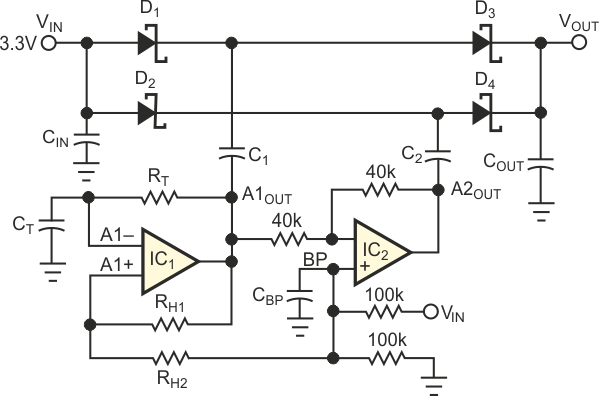Figure 2. This equivalent circuit shows the innards of the LM4871LD audio amplifier.

The following equation approximates the effective output resistance at the load: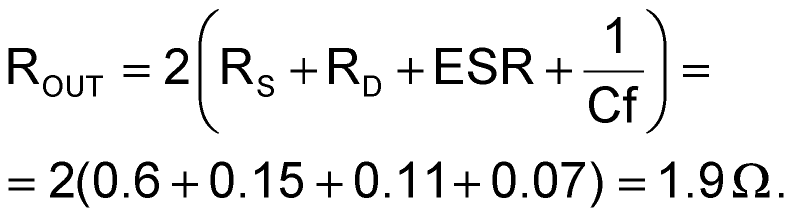Component values as shown in Figure 1 provide a circuit that can produce 5 V at 0.5 A from a 3.3 V source at a conversion efficiency of 78%. If necessary, you can obtain tighter regulation figures at slightly lower output current by adding a low-dropout linear regulator, such as the LP3961. At a 500-mA load it introduces a drop of only 150 mV. Its addition provides good line and load regulation over the range IOUT = 0 to 500 mA (Figure 3). You can also use the circuits of Figure 1 and Figure 3 to provide 3.3 V at 500 mA from a 2.5 V source.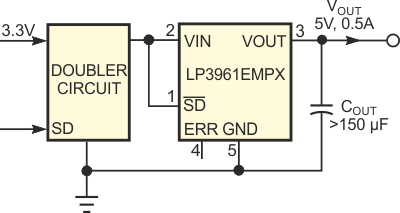Figure 3. You can tighten voltage-regulation specs in Figure 1’s circuit by adding a linear regulator.

## Materials on the topic

EDN

You may have to register before you can post comments and get full access to forum.
 User Name Remember Me? Password